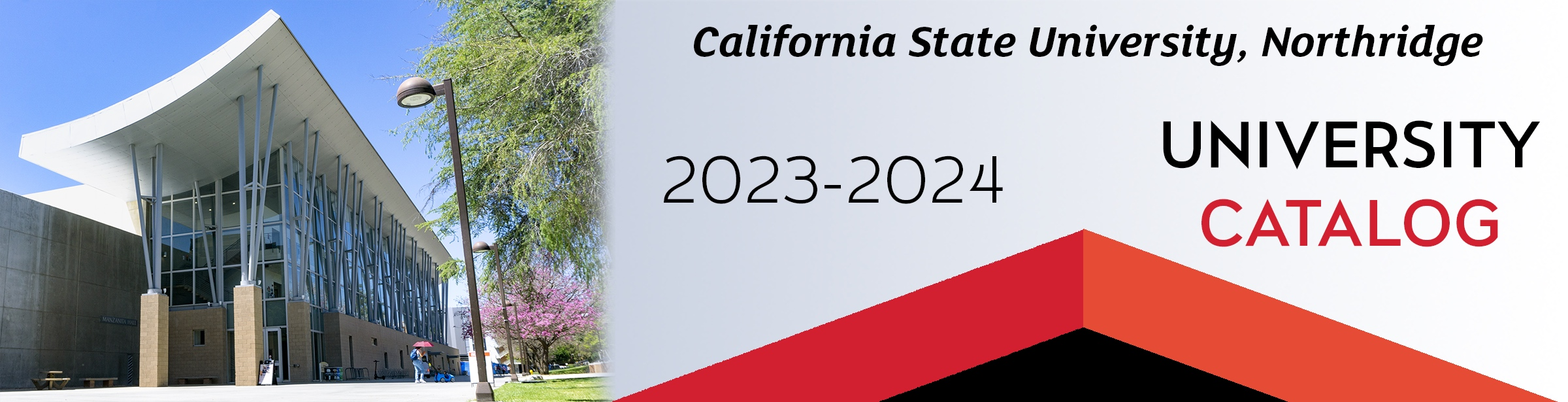## Course: ECE 280. Applied Differential Equations in Electrical Engineering (3)

Prerequisite: MATH 150B. Recommended Corequisite or Preparatory: MATH 250. Modeling of systems by ordinary differential equations. Determination of initial conditions using dynamic behavior of physical systems. Solution of ordinary differential equations by various methods, such as separation of variables, undetermined coefficients, series, and Laplace Transform. Linear algebra and solution of systems of differential equations. Numerical methods and use of application software such as MATLAB and Mathematica in solving differential equations and systems of differential equations.

### ECE 280

Class NumberLocationDayTime